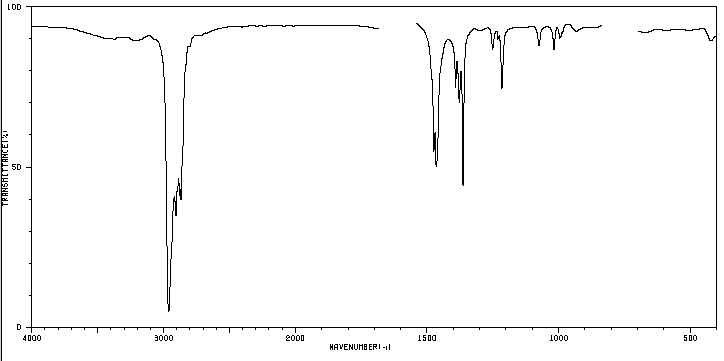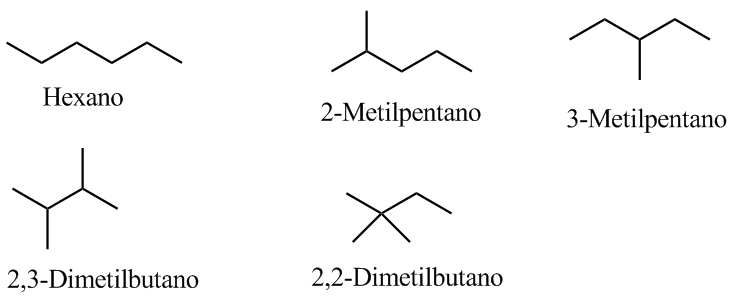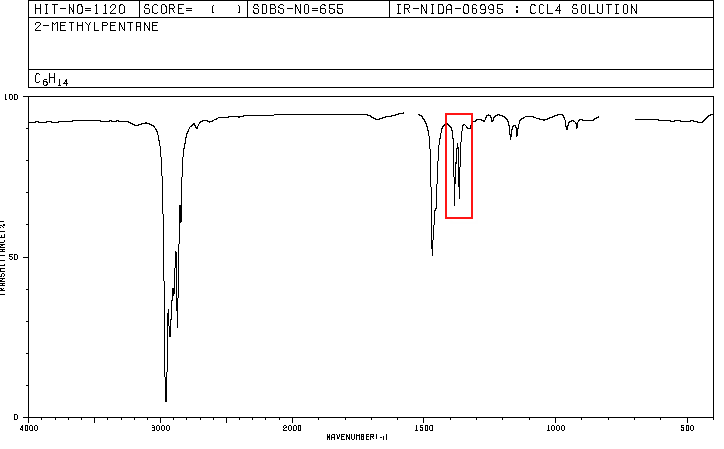An organic compound whose percentage composition is 83.63% carbon and 16.37% hydrogen with a molecular mass of 86.11, presents the following IR spectrum.Determine the structure of the compound.
1. Determination of the empirical formula
$C:\frac{83.63}{12.01}=6.96;\;\;\; H:\frac{16.37}{1.008}= 16.24$. Dividing by the smallest value $C:\frac{6.96}{6.96}=1;\;\;\;H:\frac{16.24}{6.96}=2.33$ . Multiplying by 3 we get integer values C:3 H:7. Therefore, the empirical formula is $(C_3H_7)_n$.
2. Determination of the molecular formula
$n=\frac{86.11}{3\times 12.01+7\times 1.008}=2$. The molecular formula is: C 6 H 14
3. Determination of the unsaturation index.
Comparing with the formula $C_nH_{2n+2}$ it is observed that the alkane of 6 carbons has 14 hydrogens. Therefore, our molecular formula lacks unsaturations (alkane).
4. Write the possible isomers.
Since it is a saturated compound, it will contain neither cycles nor double bonds.5. Study of the spectrum to determine which isomer it belongs to.The indicated band, due to the symmetric bending vibration of the methyl CH bonds, is split for the isopropyl and tert-butyl groups. In the case of isopropyl, two bands of equal intensity appear at 1380 and 1370 cm -1 . In the case of tert-butyl the 1380 band has half the intensity. As can be seen in our spectrum, the two bands are of different intensity, a fact that indicates the presence of a tert-butyl group in the molecule. Of the 5 isomers proposed, only one presents this group, 2,2-Dimethylbutane.
Spectra for hexane and 2-methylpentane are included below.